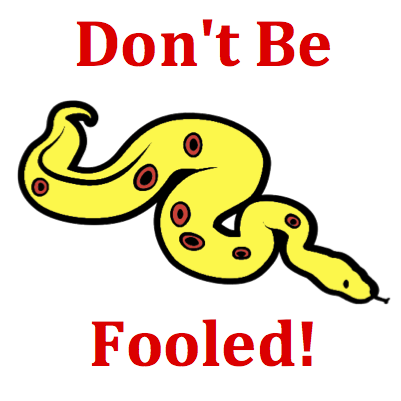# Circular and Satellite Motion - Mission CG2 Detailed HelpAn object with mass 'm' is moving in a circle of radius 'R' at a constant speed of 'v.' The acceleration of the object will INCREASE if ____. List all that apply ... .Equations are guides to assist you in thinking about relationships between variables. In this question, the relationship between mass, radius, velocity and acceleration is explored. The equation in the Formula Frenzy section shows the dependency of acceleration upon speed and radius. Clearly the acceleration is directly related to the speed and inversely related to the radius.Many students are confused by the impact of changing mass on acceleration. It would seem like mass might have an effect upon acceleration. After all, the acceleration is the net force divided by the mass. But don't be fooled! If v and R are held constant (as stated in the question), then a change in the mass will only affect the net force.The acceleration (a) of an object that is moving in a circle is dependent upon the speed (v) at which it moves and the radius (R) of the circular path about which it moves. The relationship is expressed by the following equation: a = v2​​ / R